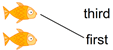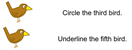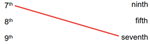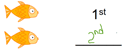# Ordinal Numbers

## Ordinal positions, numbers and words

These kindergarten math worksheets help students understand ordinal positions, numbers and words (e.g. 3rd, third).

## Match objects to their ordinal postionThese ordinal position worksheets show a sequence of  fish or birds which students match to their ordinal positions either in word form ("third") or numerical form ("3rd").In our reading ordinal numbers worksheets students are given simple instructions including ordinal numbers in word or numerical form).

## Match ordinal numbers to their wordsThese matching ordinal words and numbers worksheets require students to match ordinal numbers from 1-10 with their words.

## Write ordinal numbers and wordsIn our writing ordinal numbers and words worksheets students are asked to write the ordinal position of a series of fish; both ordinal words and numbers are used.

## More numbers worksheets

Explore all of our learning numbers worksheets (recognizing and printing numbers), counting worksheets (counting objects, skip counting, counting backwards) and comparing numbers worksheets ("more than", "less than", ordering numbers).

## What is K5?

K5 Learning offers reading and math worksheets, workbooks and an online reading and math program for kids in kindergarten to grade 5.  We help your children build good study habits and excel in school.